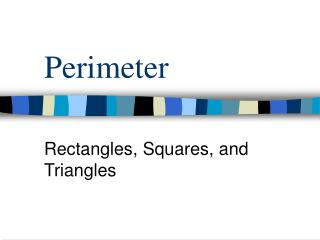DownloadDownload PresentationPerimeter

# Perimeter

Download Presentation## Perimeter

- - - - - - - - - - - - - - - - - - - - - - - - - - - E N D - - - - - - - - - - - - - - - - - - - - - - - - - - -
##### Presentation Transcript

1. Perimeter Rectangles, Squares, and Triangles

2. Perimeter • Measures the distance around the edge of any flat object. • To find the perimeter of any figure, ADD the lengths of its sides.

3. What is a rectangle? • A rectangle has: • 4 sides • 4 square corners or right angles • Opposite sides are of equal lengths • Opposite sides are directly across from each other

4. To find the perimeter of a rectangle: 18 Use the formula P= 2 x length + 2 x width P= 2 x 18 + 2 x 15 P= 36 + 30= 66 15 Or ……. You can always add the sides. P= 18 + 18 +15 +15= 66

5. What is a square? A square has: • 4 equal sides • 4 right angles

6. To find the perimeter of a square: 3 Use the formula: • P = 4 x side • P = 4 x 3 = 12 3 Or . . . . You can add the sides. P = 3 + 3 + 3 + 3 = 12

7. What is a triangle? • A triangle has 3 sides. All triangles may not look the same, but they all have 3 sides.

8. To find the perimeter of a triangle: Use the formula: • P = side + side + side • P = 6 + 6 + 4 = 16 6 6 4

9. Circumference of a Circle • The perimeter, or the distance around a circle, is called the circumference.

10. Circumference of a Circle • To find the circumference of a circle, you need to know either the diameter or radius of the circle.

11. The diameter of a circle is a line segment drawn through the center of the circle with endpoints on the circle. Diameter

12. The radius is a line segment connecting the circle’s center to a point on the circle. The length of the radius is one-half the length of the diameter. Radius Diameter

13. The formula for finding circumference (C) is C = pi x diameter. • The value of pi is about 3.14, so the formula for finding the circumference of a circle is C = 3.14 x diameter.

14. Find the circumference of the circle. • C = pi x diameter • C = 3.14 x 4= 12.56 4